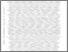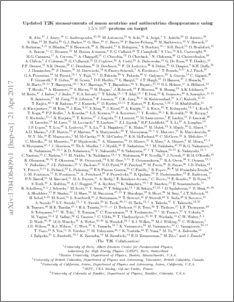# Updated T2K measurements of muon neutrino and antineutrino disappearance using 1.5 x10 21 protons on target

, T2K Collaboration (2017) Updated T2K measurements of muon neutrino and antineutrino disappearance using 1.5 x10 21 protons on target. Physical Review D, 96 (1). ISSN 1550-7998Preview
PDF (1704.06409v1)
1704.06409v1.pdf - Accepted Version

## Abstract

We report measurements by the T2K experiment of the parameters $\theta_{23}$ and $\Delta m^{2}_{32}$ governing the disappearance of muon neutrinos and antineutrinos in the three flavor neutrino oscillation model. Utilizing the ability of the experiment to run with either a mainly neutrino or a mainly antineutrino beam, the parameters are measured separately for neutrinos and antineutrinos. Using $7.482 \times 10^{20}$ POT in neutrino running mode and $7.471 \times 10^{20}$ POT in antineutrino mode, T2K obtained, $\sin^{2}(\theta_{23})=0.51^{+0.08}_{-0.07}$ and $\Delta m^{2}_{32} = 2.53^{+0.15}_{-0.13} \times 10^{-3}$eV$^{2}$/c$^{4}$ for neutrinos, and $\sin^{2}({\overline{\theta}}_{23})=0.42^{+0.25}_{-0.07}$ and ${\Delta\overline{m}^2}_{32} = 2.55^{+0.33}_{-0.27} \times 10^{-3}$eV$^{2}$/c$^{4}$ for antineutrinos (assuming normal mass ordering). No significant differences between the values of the parameters describing the disappearance of muon neutrinos and antineutrinos were observed.

Item Type:
Journal Article
Journal or Publication Title:
Physical Review D
Departments:
ID Code:
87048
Deposited By:
Deposited On:
13 Jul 2017 15:26
Refereed?:
Yes
Published?:
Published